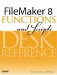# Exp()

Category: Number

 Syntax: Exp ( number )

Parameters:

numberAny expression that resolves to a numeric value.

Data type returned: Number

Description:

Returns the value of the constant e raised to the power of number. The Exp() function is the inverse of the Ln() function.

To return the value of the constant e itself, use Exp (1), which returns 2.7182818284590452. You can use the SetPrecision() function to return e with up to 400 digits of precision.

Examples:

Function

Results

Round ( Exp ( 5 ); 3 )

Returns 148.413.

Exp ( Ln ( 5 ))

Returns 5.FileMaker 8 Functions and Scripts Desk Reference
ISBN: 0789735113
EAN: 2147483647
Year: 2004
Pages: 352